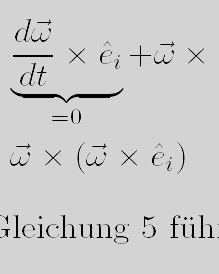home

### Miscellaneous material (mostly math and physics related)

1. A derivation of the infamous coriolis force (in German) (pdf) and (.tex)
2. A short introduction to (linear) differential equations (in German) (pdf) and (.tar)
3. A introduction to Bayesian statistics (in German) (pdf) and (.tar)
4. Exercises (and solutions) for an introductionary physics course (in German) (pdf)
5. A quick introduction into calculus and Taylor expansion (in German) (pdf) and (.tar)
6. A very brief survey on symmetry and symmetry-breaking in particle physics (in German) (pdf) and (.tex)
7. Two pages on how one gets from the Lagrange function to the Lagrange density (in German) (pdf) and (.tex)
8. Well, it is not exactly science but a neat application of the geometric series: the formula for compound interest (in German) (pdf) and (.tex)